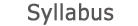2019 - 2020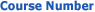0509-2804-11
Numerical Analysis
FACULTY OF ENGINEERING | DEPARTMENTS OF ENGINEERING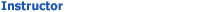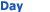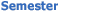Ido Hadi Engineering Studies - Classrooms 207 Wed 1800-1900 Sem  2
Course description
Credit Points: 3.5
Prerequisites: Programming; Ordinary Differential Equations
Number systems and errors. Integers. Representation of fractions. Floating point arithmetic. Error propagation. Interpolation by polynomial. Newton's method. Uniqueness of the interpolating polynomial. Lagrange interpolation. Divided differences table. Error estimation. Approximation methods: basic concepts of functional analysis (linear space, norm, inner product). Approximation by polynomials and orthogonal polynomials. Least squares approximations. Fourier series. FFT. Piecewise polynomial approximations. Splines. Matrices and systems of linear equations. Elimination, pivoting, factorization. Norms. Iterative methods and approximations. Numerical stability and condition number. Determinants. Eigenvalue problems. Stabilization methods, SVD and least square solutions. Numerical differentiation and integration. Error estimation. The trapezoid, Newton, and Gauss methods. Romberg integration. Comparison of the methods. Ordinary differential equations. Elementary finite differences methods and Taylor series. One step methods. Runge-Kutta method. Predictor-Corrector method. Convergence and stability. Systems of ODE's. Boundary value problems. Nonlinear equations. Iterative methods. Fixed-point theorem. Newton method. The gradient method.

accessibility declaration